# Excel DEGREES Function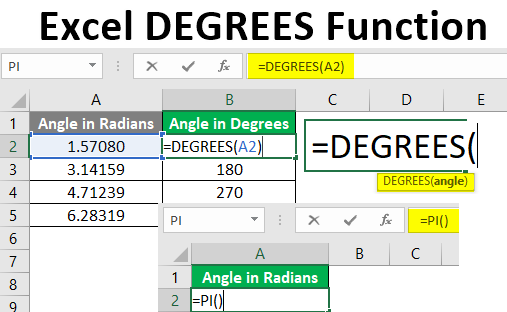• Introduction to DEGREES Function
• How to Use DEGREES Function in Excel?

## Introduction to DEGREES Function

The Degrees Function in Excel is one of the simplest functions to be used. It belongs to the category of “Math and Trigonometry function” in Excel. The function converts an angle value, which it takes as a parameter, from radians to degrees. The function was introduced in MS Excel 2000 edition. The function proves handy in situations that require use of angle values presented in degrees. The function has a very simple syntax which is as shown below.

Syntax: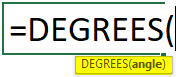Here, the angle is represented in radians, and the DEGREES function successfully converts it into corresponding degree values.

### How to Use DEGREES Function in Excel?

The mathematical relation between two units of angle that we saw in the above section does allow us to determine value in degrees for any angle value. However, Excel provides us DEGREES function using which we can easily find the corresponding value in degrees. The following section shows the implementation of the DEGREES function in Excel. Let’s go through the following steps.

You can download this Excel DEGREES Function Template here – Excel DEGREES Function Template

We have a table containing angle values. We intend to convert these values from radians to degrees. First, have a look at the data. On the left-hand side column, we have four angle values represented in radians, and on the right-hand side, we have angle values presented in degrees.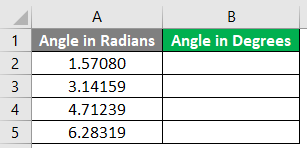As we intend to convert these values from radians to degrees, we shall implement the DEGREES function. When we start typing the name of the function, Excel automatically pops-up the names of all the functions for which the names start from D. We can select the DEGREES function by moving to using arrow keys or by just clicking on it. As we can see, the function description reads “Converts radians to degrees”. So, Excel helps us to understand the DEGREES function by providing the most appropriate definition.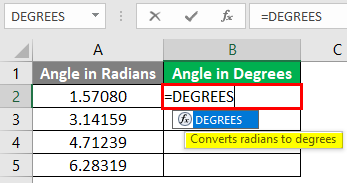When we type the opening bracket, Excel highlights the argument which the function takes in order to perform its function. Here, in case of the DEGREES function, we have only one argument which is angle. Go through the following screenshot.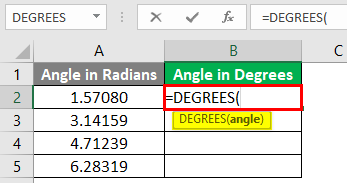To convert the corresponding angle from radians to degrees, we passed the value as illustrated in the below screenshot. As we can see, we passed the cell containing requisite angle value in radians, as the parameter in the DEGREES function.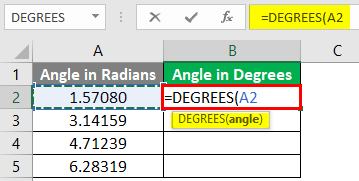Now, observe the result that we got as can be seen in the following table. As we can see, the DEGREES function has given us the correct result i.e. 90. So, this means that 1.57080 radians are equal to 90 degrees. In a similar way, we shall convert other angle values also from radians to degrees.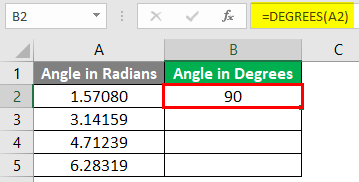We just copied the function down across all the required cells, and got correct conversions of angle values from radians to degrees. The following table shows the angle values in radians and the corresponding angle values in degrees obtained using the DEGREES function. Likewise, we can convert any angle value from radians to degrees.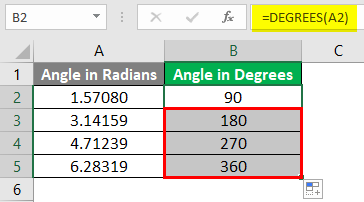The formula implemented in the above table is as follows. Go through the table and see how the DEGREES function takes the argument.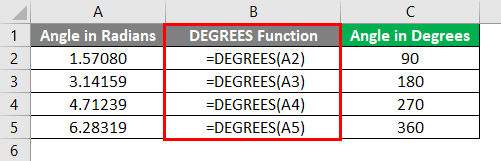In the earlier part, we saw the importance of ∏ in conversion of angle from radians to degrees. In Excel, we have a function which is called PI(). This function gives a constant value i.e. 3.14159. We can work with DEGREES function using this function also. Let’s see this as illustrated below.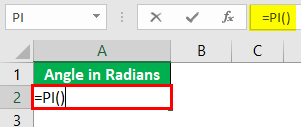After using PI Formula, the output shown in below.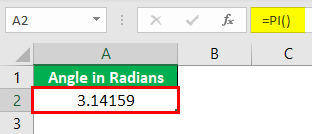Using DEGREES Function in cell A2.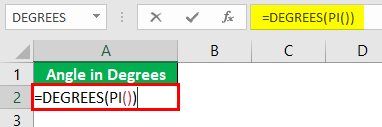After using the above formula, the output is shown below.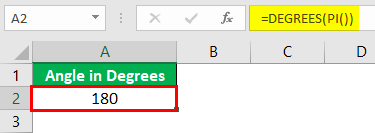Going through the above screenshot, we can see how PI() function can be passed as a parameter into the DEGREES function. Using the PI() function, we can avoid the direct use of direct values. The PI() function can be divided as a suitable constant and then can be passed as a parameter in the DEGREES function. So, there are various ways to work with and use the DEGREES function, and the best-suited method should be employed for use.

We can also pass a negative value in the DEGREES function. Negative angles just indicate a different direction of the way in which angles are measured. The following table shows how the DEGREES function returned the appropriate values for the negative angles too.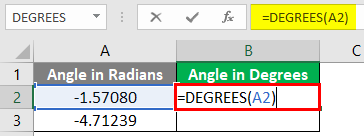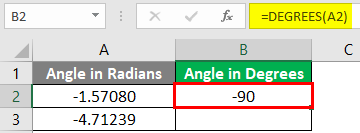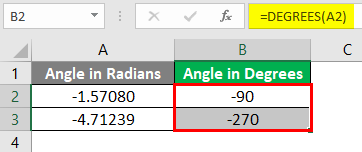We will see the basics of angle conversion from radians to degrees.

• In geometry, radians and degrees represent the units i.e. various ways of representing the measurement of the angle which is subtended at the center of the circle by an arc. In order to understand how the conversion from radians to degrees happens for angles, we must know the relation between the two units. The relation can be written as shown below.

• ∏ is a constant which has a value of 3.14159. So 180 degrees amount to 3.14159 radians. Using this relation we can calculate a value in degrees for any angle which is represented in radians. So, we have 1 radian equal to about 57.3 degrees. And if we want to find value for 270 degrees, the formula shall be (3.14159 X 270) / 180. The value thus obtained would be in degrees. This formula can be written as (∏ X 270)/180.

### Things to Remember About Excel DEGREES Function

• While Excel provides a self-explanatory approach for use of its every functionality and the implementation of the DEGREES function is easy.
• Valid parameters must be passed into the function or else, the function shall return an error.
• Though the function looks simple, it is a context-based function which is used in situations that involve complex mathematical problems and calculations.
• In such cases amongst all the options available, the DEGREES function is the best one.

### Recommended Articles

This has been a guide to Excel DEGREES Function. Here we discuss how to use Excel DEGREES Function along with practical examples and downloadable excel template. You can also go through our other suggested articles –

1. Compare Two Lists in Excel
2. Excel Evaluate Formula
3. Excel Text with Formula
4. Timecard Template in Excel

The post Excel DEGREES Function appeared first on EDUCBA.

This post first appeared on Best Online Training & Video Courses | EduCBA, please read the originial post: here